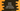# Kotlin if-else expression explanation with examples# Introduction :

if-else is used in almost all programming languages. These are basic control statements and we can control the programming flow based on a condition. Similar to any other programming language, we can use if-else in kotlin with a condition. In kotlin, we can also use if-else as an expression i.e. if-else returns a value!

In this blog post, I will show you a couple of different examples on how to use if-else, if-elseif-else, nested if-else in Kotlin like a traditional way and like an expression.

## Kotlin if-else example :

The basic syntax of if-else is :

``````if (condition){
//code for if block
}else{
//code for else block
}``````

If you run an if-else block like the above one, it will check the condition expression first. If it is true, it will execute the code written in the if block. If the condition is false, it will execute the code written in the else block.

For example :

``````fun main(args: Array<string>) {
print("Enter a number :")

if(n%2 == 0){
print("\$n is an even number\n")
}else{
print("\$n is an odd number\n")
}
}``````

Sample outputs :

``````Enter a number :12
12 is an even number

Enter a number :13
13 is an odd number``````

### Kotlin way :

I have mentioned above that we can use if-else as an expression in Kotlin. We can write the above program as like below :

``````fun main(args: Array<string>) {
print("Enter a number :")

val result = if(n%2 == 0){
"\$n is an even number\n"
}else{
"\$n is an odd number\n"
}

print(result)
}``````

It will give the same output.

Here, the if-else block returns one string and we are assigning this value to the val result.

## if-else if-else example :

if-elseif-else is similar to if-else. The only change is that it adds one extra condition elseif. The program first checks the condition defined in the if block. If it is false, it checks the elseif block and finally else block.

``````fun main(args: Array<string>) {
print("Enter a number :")

if (n%2 == 0) {
print("\$n is divisible by 2\n")
}else if (n%3 == 0) {
print("\$n is divisible by 3\n")
}else{
print("Not sure !!\n")
}
}``````

### Kotlin way :

Similar to the above example, we can change the implementation of this program as like below :

``````fun main(args: Array<string>) {
print("Enter a number :")

val result = if (n%2 == 0) {
"\$n is divisible by 2\n"
}else if (n%3 == 0) {
"\$n is divisible by 3\n"
}else{
"Not sure !!\n"
}

print(result)
}``````

As explained above, the if-elseif-else block also returns one value.

## Nested if-else expression :

Nested if-else expressions are expressions that contain if-else expression inside another if-else expression.

In Kotlin, we can use nested if-else expressions as like below :

``````fun main(args: Array<string>) {
print("Enter a number :")

val result = if (n>0) {
if (n%5 == 0) {
"\$n is divisible by 5\n"
}else{
"\$n is not divisible by 5\n"
}
}else{
}

print(result)
}``````

This program checks if the user input number is positive and divisible by 5 or not. If it is negative, it prints one message. The final string is saved in the result variable and result is printed at the end of the program.

Sample output :

``````Enter a number :12
12 is not divisible by 5

Enter a number :-12